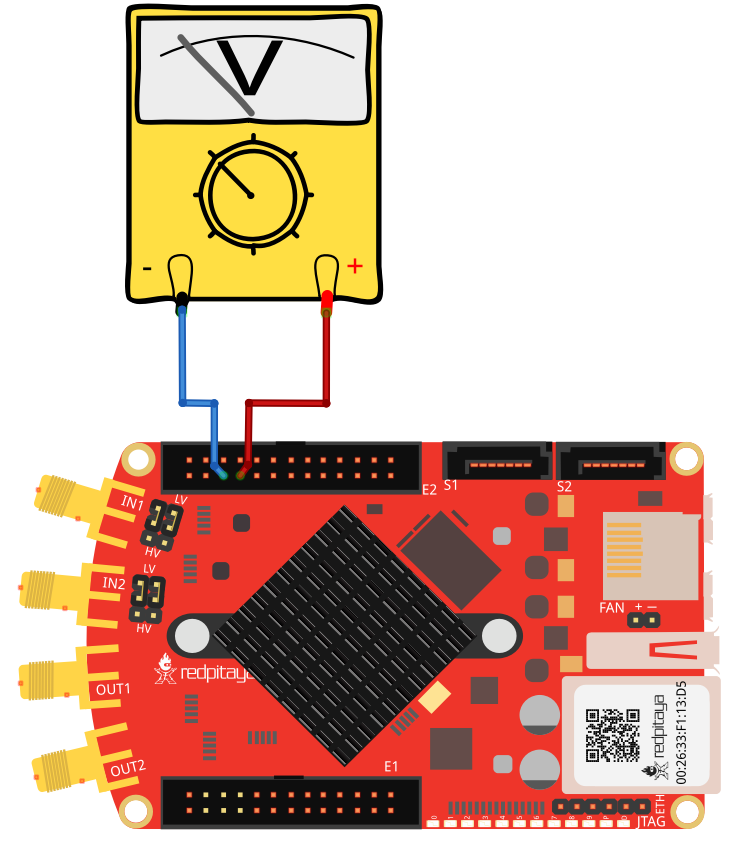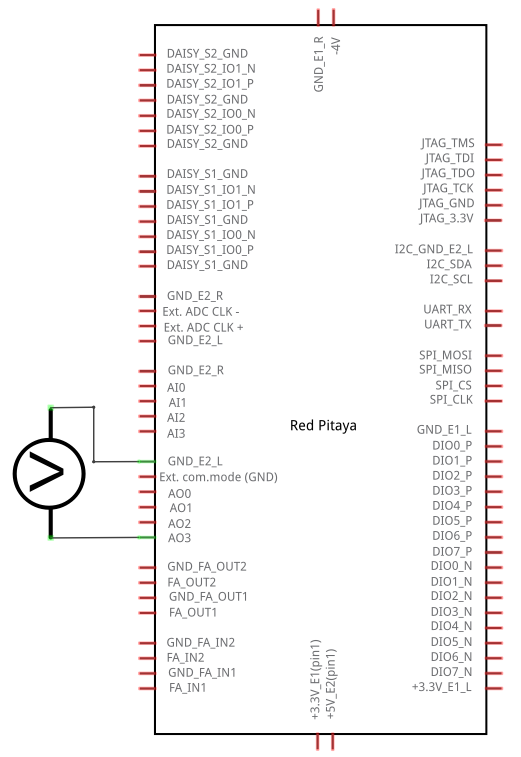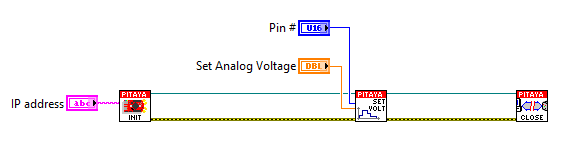# 2.3.1.4.2.3. Interactive voltage setting on slow analog output¶

## 2.3.1.4.2.3.1. Description¶

This example shows how to set analog voltage on slow analog Red Pitaya outputs using MATLAB slider. Slow analog outputs on Red Pitaya are in range from 0 to 1.8 Volts.

## 2.3.1.4.2.3.2. Required hardware¶

• Red Pitaya device

• Voltmeter

Wiring example for STEMlab 125-14 & STEMlab 125-10:## 2.3.1.4.2.3.3. Circuit¶## 2.3.1.4.2.3.4. Code - MATLAB®¶

The code is written in MATLAB. In the code we use SCPI commands and TCP/IP communication. Copy code from below to MATLAB editor, save project and press run.

```function sliderDemo

f = figure(1);
global p

%// initialize the slider
h = uicontrol(...
'parent'  , f,...
'units'   , 'normalized',...     %// pixels settings
'style'   , 'slider',...
'position', [0.05 0.05 0.9 0.05],...
'min'     , 1,...                %// Make the "value" between min ...
'max'     , 100,...              %// max 10, with initial value
'value'   , 10,...               %// as set.
'callback', @sliderCallback);    %// This is called when using the
%// arrows
%// and/or when clicking the slider bar

%// (variable appears unused, but not assigning it to anything means that
%// the listener is stored in the 'ans' variable. If "ans" is overwritten,
%// the listener goes out of scope and is thus destroyed, and thus, it no
%// longer works.

function  sliderCallback(~,~)

p =(get(h,'value'))

%% Define Red Pitaya as TCP/IP object

IP= '192.168.178.108';           % Input IP of your Red Pitaya...
port = 5000;
tcpipObj=tcpip(IP, port);

%% Open connection with your Red Pitaya

fopen(tcpipObj);
tcpipObj.Terminator = 'CR/LF';

%% Set your output voltage value and pin

out_voltage = num2str((1.8/100)*p)      % From 0 - 1.8 volts
out_num = '2';                          % Analog outputs 0,1,2,3
%% Set your SCPI command with strcat function

scpi_command = strcat('ANALOG:PIN AOUT',out_num,',',out_voltage);

%% Send SCPI command to Red Pitaya

fprintf(tcpipObj,scpi_command);

%% Close connection with Red Pitaya

fclose(tcpipObj);
end
end
```

## 2.3.1.4.2.3.5. Code - LabVIEW¶